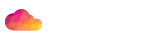# #NAME

ojo - Fun one-liners with Mojo

# #SYNOPSIS

``\$ perl -Mojo -E 'say g("mojolicious.org")->dom->at("title")->text'``

# #DESCRIPTION

A collection of automatically exported functions for fun Perl one-liners. Ten redirects will be followed by default, you can change this behavior with the `MOJO_MAX_REDIRECTS` environment variable.

``\$ MOJO_MAX_REDIRECTS=0 perl -Mojo -E 'say g("example.com")->code'``

Proxy detection is enabled by default, but you can disable it with the `MOJO_PROXY` environment variable.

``\$ MOJO_PROXY=0 perl -Mojo -E 'say g("example.com")->body'``

TLS certificate verification can be disabled with the `MOJO_INSECURE` environment variable.

``\$ MOJO_INSECURE=1 perl -Mojo -E 'say g("https://127.0.0.1:3000")->body'``

Every ojo one-liner is also a Mojolicious::Lite application.

``\$ perl -Mojo -E 'get "/" => {inline => "%= time"}; app->start' get /``

On Perl 5.20+ subroutine signatures will be enabled automatically.

``\$ perl -Mojo -E 'a(sub (\$c) { \$c->render(text => "Hello!") })->start' get /``

If it is not already defined, the `MOJO_LOG_LEVEL` environment variable will be set to `fatal`.

# #FUNCTIONS

ojo implements the following functions, which are automatically exported.

## #a

``my \$app = a('/hello' => sub { \$_->render(json => {hello => 'world'}) });``

Create a route with "any" in Mojolicious::Lite and return the current Mojolicious::Lite object. The current controller object is also available to actions as `\$_`. See also Mojolicious::Guides::Tutorial for more argument variations.

``\$ perl -Mojo -E 'a("/hello" => {text => "Hello Mojo!"})->start' daemon``

## #b

``my \$stream = b('lalala');``

Turn string into a Mojo::ByteStream object.

``\$ perl -Mojo -E 'b(g("mojolicious.org")->body)->html_unescape->say'``

## #c

``my \$collection = c(1, 2, 3);``

Turn list into a Mojo::Collection object.

## #d

``````my \$res = d('example.com');
my \$res = d('http://example.com' => {Accept => '*/*'} => 'Hi!');
my \$res = d('http://example.com' => {Accept => '*/*'} => form => {a => 'b'});
my \$res = d('http://example.com' => {Accept => '*/*'} => json => {a => 'b'});``````

Perform `DELETE` request with "delete" in Mojo::UserAgent and return resulting Mojo::Message::Response object.

## #f

``my \$path = f('/home/sri/foo.txt');``

Turn string into a Mojo::File object.

``\$ perl -Mojo -E 'say r j f("hello.json")->slurp'``

## #g

``````my \$res = g('example.com');
my \$res = g('http://example.com' => {Accept => '*/*'} => 'Hi!');
my \$res = g('http://example.com' => {Accept => '*/*'} => form => {a => 'b'});
my \$res = g('http://example.com' => {Accept => '*/*'} => json => {a => 'b'});``````

Perform `GET` request with "get" in Mojo::UserAgent and return resulting Mojo::Message::Response object.

``\$ perl -Mojo -E 'say g("mojolicious.org")->dom("h1")->map("text")->join("\n")'``

## #h

``````my \$res = h('example.com');
my \$res = h('http://example.com' => {Accept => '*/*'} => 'Hi!');
my \$res = h('http://example.com' => {Accept => '*/*'} => form => {a => 'b'});
my \$res = h('http://example.com' => {Accept => '*/*'} => json => {a => 'b'});``````

Perform `HEAD` request with "head" in Mojo::UserAgent and return resulting Mojo::Message::Response object.

## #j

``````my \$bytes = j([1, 2, 3]);
my \$bytes = j({foo => 'bar'});
my \$value = j(\$bytes);``````

Encode Perl data structure or decode JSON with "j" in Mojo::JSON.

``\$ perl -Mojo -E 'f("hello.json")->spurt(j {hello => "world!"})'``

## #n

``````n {...};
n {...} 100;``````

Benchmark block and print the results to `STDERR`, with an optional number of iterations, which defaults to `1`.

``\$ perl -Mojo -E 'n { say g("mojolicious.org")->code }'``

## #o

``````my \$res = o('example.com');
my \$res = o('http://example.com' => {Accept => '*/*'} => 'Hi!');
my \$res = o('http://example.com' => {Accept => '*/*'} => form => {a => 'b'});
my \$res = o('http://example.com' => {Accept => '*/*'} => json => {a => 'b'});``````

Perform `OPTIONS` request with "options" in Mojo::UserAgent and return resulting Mojo::Message::Response object.

## #p

``````my \$res = p('example.com');
my \$res = p('http://example.com' => {Accept => '*/*'} => 'Hi!');
my \$res = p('http://example.com' => {Accept => '*/*'} => form => {a => 'b'});
my \$res = p('http://example.com' => {Accept => '*/*'} => json => {a => 'b'});``````

Perform `POST` request with "post" in Mojo::UserAgent and return resulting Mojo::Message::Response object.

## #r

``my \$perl = r({data => 'structure'});``

Dump a Perl data structure with "dumper" in Mojo::Util.

``perl -Mojo -E 'say r g("example.com")->headers->to_hash'``

## #t

``````my \$res = t('example.com');
my \$res = t('http://example.com' => {Accept => '*/*'} => 'Hi!');
my \$res = t('http://example.com' => {Accept => '*/*'} => form => {a => 'b'});
my \$res = t('http://example.com' => {Accept => '*/*'} => json => {a => 'b'});``````

Perform `PATCH` request with "patch" in Mojo::UserAgent and return resulting Mojo::Message::Response object.

## #u

``````my \$res = u('example.com');
my \$res = u('http://example.com' => {Accept => '*/*'} => 'Hi!');
my \$res = u('http://example.com' => {Accept => '*/*'} => form => {a => 'b'});
my \$res = u('http://example.com' => {Accept => '*/*'} => json => {a => 'b'});``````

Perform `PUT` request with "put" in Mojo::UserAgent and return resulting Mojo::Message::Response object.

## #x

``my \$dom = x('<div>Hello!</div>');``

Turn HTML/XML input into Mojo::DOM object.

``\$ perl -Mojo -E 'say x(f("test.html")->slurp)->at("title")->text'``